# 10   Feature Based Grammar

## 10.1   Introduction

Imagine you are building a spoken dialogue system to answer queries about train schedules in Europe. (1) illustrates one of the input sentences that the system should handle.

 (1) Which stations does the 9.00 express from Amsterdam to Paris stop at?

The information that the customer is seeking is not exotic — the system back-end just needs to look up the list of stations on the route, and reel them off. But you have to be careful in giving the correct semantic interpretation to (1). You don't want to end up with the system trying to answer (2) instead:

 (2) Which station does the 9.00 express from Amsterdam terminate at?

Part of your solution might use domain knowledge to figure out that if a speaker knows that the train is a train to Paris, then she probably isn't asking about the terminating station in (1). But the solution will also involve recognizing the syntactic structure of the speaker's query. In particular, your analyzer must recognize that there is a syntactic connection between the question phrase which stations, and the phrase stop at at the end (1). The required interpretation is made clearer in the "quiz question version shown in (3), where the question phrase fills the "gap" that is implicit in (1):

 (3) The 9.00 express from Amsterdam to Paris stops at which stations?

 (4)

The only way of telling which noun phrase is the subject of kennen ('know') and which is the object is by looking at the agreement inflection on the verb — word order is no help to us here. Since verbs in German agree in number with their subjects, the plural form kennen requires Welche Studenten as subject, while the singular form kennt requires Franz as subject. The fact that subjects and verbs must agree in number can be expressed within the CFGs that we presented in Chapter 7. But capturing the fact that the interpretations of germanagra and germanagrb differ is more challenging. In this chapter, we will only examine the syntactic aspect of local dependencies such as number agreement. In Chapter 11, we will demonstrate how feature-based grammars can be extended so that they build a representation of meaning in parallel with a representation of syntactic structure.

Note

Remember that our program samples assume you begin your interactive session or your program with: import nltk, re, pprint

## 10.2   Why Features?

We have already used the term "feature" a few times, without saying what it means. What's special about feature-based grammars? The core ideas are probably already familiar to you. To make things concrete, let's look at the simple phrase these dogs. It's composed of two words. We'll be a bit abstract for the moment, and call these words a and b. We'll be modest, and assume that we do not know everything about them, but we can at least give a partial description. For example, we know that the orthography of a is these, its phonological form is DH IY Z, its part-of-speech is Det, and its number is plural. We can use dot notation to record these observations:

 (5) ```a.spelling = these a.phonology = DH IY Z a.pos = Det a.number = plural ```

Thus (5) is a partial description of a word; it lists some attributes, or features, of the word, and declares their values. There are other attributes that we might be interested in, which have not been specified; for example, what head the word is dependent on (using the notion of dependency discussed in Chapter 7), and what the lemma of the word is. But this omission of some attributes is exactly what you would expect from a partial description!

We will start off this chapter by looking more closely at the phenomenon of syntactic agreement; we will show how agreement constraints can be expressed elegantly using features, and illustrate their use in a simple grammar. Feature structures are a general data structure for representing information of any kind; we will briefly look at them from a more formal point of view, and explain how to create feature structures in Python. In the final part of the chapter, we demonstrate that the additional expressiveness of features opens out a wide spectrum of possibilities for describing sophisticated aspects of linguistic structure.

### 10.2.1   Syntactic Agreement

Consider the following contrasts:

(6)

 a. this dog

 b. *these dog

(7)

 a. these dogs

 b. *this dogs

In English, nouns are usually morphologically marked as being singular or plural. The form of the demonstrative also varies: this (singular) and these (plural). Examples (6b) and (7b) show that there are constraints on the use of demonstratives and nouns within a noun phrase: either both are singular or both are plural. A similar constraint holds between subjects and predicates:

(8)

 a. the dog runs

 b. *the dog run

(9)

 a. the dogs run

 b. *the dogs runs

Here we can see that morphological properties of the verb co-vary with syntactic properties of the subject noun phrase. This co-variance is called agreement. If we look further at verb agreement in English, we will see that present tense verbs typically have two inflected forms: one for third person singular, and another for every other combination of person and number:

Table 10.1:

Agreement Paradigm for English Regular Verbs

 singular plural 1st per I run we run 2nd per you run you run 3rd per he/she/it runs they run

We can make the role of morphological properties a bit more explicit as illustrated in runs and run. These representations indicate that the verb agrees with its subject in person and number. (We use "3" as an abbreviation for 3rd person, "SG" for singular and "PL" for plural.)

Let's see what happens when we encode these agreement constraints in a context-free grammar. We will begin with the simple CFG in (10).

 (10) ```s → np vp np → Det n vp → v Det → 'this' n → 'dog' v → 'runs' ```

Example (10) allows us to generate the sentence this dog runs; however, what we really want to do is also generate these dogs run while blocking unwanted strings such as *this dogs run and *these dog runs. The most straightforward approach is to add new non-terminals and productions to the grammar:

 (11) ```S_SG → NP_SG VP_SG S_PL → NP_PL VP_PL NP_SG → Det_SG N_SG NP_PL → Det_PL N_PL VP_SG → V_SG VP_PL → V_PL Det_SG → 'this' Det_PL → 'these' N_SG → 'dog' N_PL → 'dogs' V_SG → 'runs' V_PL → 'run' ```

It should be clear that this grammar will do the required task, but only at the cost of duplicating our previous set of productions.

### 10.2.2   Using Attributes and Constraints

We spoke informally of linguistic categories having properties; for example, that a noun has the property of being plural. Let's make this explicit:

 (12) ```N[num=pl] ```

In (12), we have introduced some new notation which says that the category N has a feature called num (short for 'number') and that the value of this feature is pl (short for 'plural'). We can add similar annotations to other categories, and use them in lexical entries:

 (13) ```Det[num=sg] → 'this' Det[num=pl] → 'these' N[num=sg] → 'dog' N[num=pl] → 'dogs' V[num=sg] → 'runs' V[num=pl] → 'run' ```

Does this help at all? So far, it looks just like a slightly more verbose alternative to what was specified in (11). Things become more interesting when we allow variables over feature values, and use these to state constraints:

(14)

 a. ```S → NP[num=?n] VP[num=?n] ```

 b. ```NP[num=?n] → Det[num=?n] N[num=?n] ```

 c. ```VP[num=?n] → V[num=?n] ```

We are using "?n" as a variable over values of num; it can be instantiated either to sg or pl. Its scope is limited to individual productions. That is, within (14a), for example, ?n must be instantiated to the same constant value; we can read the production as saying that whatever value NP takes for the feature num, VP must take the same value.

In order to understand how these feature constraints work, it's helpful to think about how one would go about building a tree. Lexical productions will admit the following local trees (trees of depth one):

(15)

 a.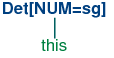b.(16)

 a.b.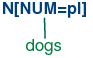Now (14b) says that whatever the num values of N and Det are, they have to be the same. Consequently, (14b) will permit (15a) and (16a) to be combined into an NP as shown in (17a) and it will also allow (15b) and (16b) to be combined, as in (17b). By contrast, (18a) and (18b) are prohibited because the roots of their constituent local trees differ in their values for the num feature.

(17)

 a.b.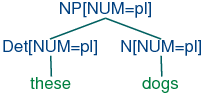(18)

 a.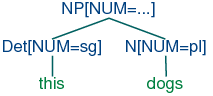b.Production (14c) can be thought of as saying that the num value of the head verb has to be the same as the num value of the VP mother. Combined with (14a), we derive the consequence that if the num value of the subject head noun is pl, then so is the num value of the VP's head verb.

 (19)The grammar in listing 10.1 illustrates most of the ideas we have introduced so far in this chapter, plus a couple of new ones.

 ```>>> nltk.data.show_cfg('grammars/feat0.fcfg') % start S # ############################ # Grammar Rules # ############################ # S expansion rules S -> NP[NUM=?n] VP[NUM=?n] # NP expansion rules NP[NUM=?n] -> N[NUM=?n] NP[NUM=?n] -> PropN[NUM=?n] NP[NUM=?n] -> Det[NUM=?n] N[NUM=?n] NP[NUM=pl] -> N[NUM=pl] # VP expansion rules VP[TENSE=?t, NUM=?n] -> IV[TENSE=?t, NUM=?n] VP[TENSE=?t, NUM=?n] -> TV[TENSE=?t, NUM=?n] NP # ############################ # Lexical Rules # ############################ Det[NUM=sg] -> 'this' | 'every' Det[NUM=pl] -> 'these' | 'all' Det -> 'the' | 'some' PropN[NUM=sg]-> 'Kim' | 'Jody' N[NUM=sg] -> 'dog' | 'girl' | 'car' | 'child' N[NUM=pl] -> 'dogs' | 'girls' | 'cars' | 'children' IV[TENSE=pres, NUM=sg] -> 'disappears' | 'walks' TV[TENSE=pres, NUM=sg] -> 'sees' | 'likes' IV[TENSE=pres, NUM=pl] -> 'disappear' | 'walk' TV[TENSE=pres, NUM=pl] -> 'see' | 'like' IV[TENSE=past, NUM=?n] -> 'disappeared' | 'walked' TV[TENSE=past, NUM=?n] -> 'saw' | 'liked'```

Listing 10.1 (feat0cfg.py): Example Feature-Based Grammar

Notice that a syntactic category can have more than one feature; for example, v[tense=pres, num=pl]. In general, we can add as many features as we like.

Notice also that we have used feature variables in lexical entries as well as grammatical productions. For example, the has been assigned the category Det[num=?n]. Why is this? Well, you know that the definite article the can combine with both singular and plural nouns. One way of describing this would be to add two lexical entries to the grammar, one each for the singular and plural versions of the. However, a more elegant solution is to leave the num value underspecified and letting it agree in number with whatever noun it combines with.

A final detail about 10.1 is the statement %start S. This a "directive" that tells the parser to take s as the start symbol for the grammar.

In general, when we are trying to develop even a very small grammar, it is convenient to put the productions in a file where they can be edited, tested and revised. We have saved 10.1 as a file named 'feat0.fcfg' in the NLTK data distribution, and it can be accessed using nltk.data.load().

We can inspect the productions and the lexicon using the commands print g.earley_grammar() and pprint(g.earley_lexicon()).

Next, we can tokenize a sentence and use the nbest_parse() function to invoke the Earley chart parser.

 ```>>> tokens = 'Kim likes children'.split() >>> from nltk.parse import load_earley >>> cp = load_earley('grammars/feat0.fcfg', trace=2) >>> trees = cp.nbest_parse(tokens) |.K.l.c.| Processing queue 0 Predictor |> . . .| [0:0] S[] -> * NP[NUM=?n] VP[NUM=?n] {} Predictor |> . . .| [0:0] NP[NUM=?n] -> * N[NUM=?n] {} Predictor |> . . .| [0:0] NP[NUM=?n] -> * PropN[NUM=?n] {} Predictor |> . . .| [0:0] NP[NUM=?n] -> * Det[NUM=?n] N[NUM=?n] {} Predictor |> . . .| [0:0] NP[NUM='pl'] -> * N[NUM='pl'] {} Scanner |[-] . .| [0:1] 'Kim' Scanner |[-] . .| [0:1] PropN[NUM='sg'] -> 'Kim' * Processing queue 1 Completer |[-] . .| [0:1] NP[NUM='sg'] -> PropN[NUM='sg'] * Completer |[-> . .| [0:1] S[] -> NP[NUM=?n] * VP[NUM=?n] {?n: 'sg'} Predictor |. > . .| [1:1] VP[NUM=?n, TENSE=?t] -> * IV[NUM=?n, TENSE=?t] {} Predictor |. > . .| [1:1] VP[NUM=?n, TENSE=?t] -> * TV[NUM=?n, TENSE=?t] NP[] {} Scanner |. [-] .| [1:2] 'likes' Scanner |. [-] .| [1:2] TV[NUM='sg', TENSE='pres'] -> 'likes' * Processing queue 2 Completer |. [-> .| [1:2] VP[NUM=?n, TENSE=?t] -> TV[NUM=?n, TENSE=?t] * NP[] {?n: 'sg', ?t: 'pres'} Predictor |. . > .| [2:2] NP[NUM=?n] -> * N[NUM=?n] {} Predictor |. . > .| [2:2] NP[NUM=?n] -> * PropN[NUM=?n] {} Predictor |. . > .| [2:2] NP[NUM=?n] -> * Det[NUM=?n] N[NUM=?n] {} Predictor |. . > .| [2:2] NP[NUM='pl'] -> * N[NUM='pl'] {} Scanner |. . [-]| [2:3] 'children' Scanner |. . [-]| [2:3] N[NUM='pl'] -> 'children' * Processing queue 3 Completer |. . [-]| [2:3] NP[NUM='pl'] -> N[NUM='pl'] * Completer |. [---]| [1:3] VP[NUM='sg', TENSE='pres'] -> TV[NUM='sg', TENSE='pres'] NP[] * Completer |[=====]| [0:3] S[] -> NP[NUM='sg'] VP[NUM='sg'] * Completer |[=====]| [0:3] [INIT][] -> S[] *```

Listing 10.2 (featurecharttrace.py): Trace of Feature-Based Chart Parser

Observe that the parser works directly with the underspecified productions given by the grammar. That is, the Predictor rule does not attempt to compile out all admissible feature combinations before trying to expand the non-terminals on the left hand side of a production. However, when the Scanner matches an input word against a lexical production that has been predicted, the new edge will typically contain fully specified features; e.g., the edge [PropN[num = sg] → 'Kim', (0, 1)]. Recall from Chapter 7 that the Fundamental (or Completer) Rule in standard CFGs is used to combine an incomplete edge that's expecting a nonterminal B with a following, complete edge whose left hand side matches B. In our current setting, rather than checking for a complete match, we test whether the expected category B will unify with the left hand side B' of a following complete edge. We will explain in more detail in Section 10.3 how unification works; for the moment, it is enough to know that as a result of unification, any variable values of features in B will be instantiated by constant values in the corresponding feature structure in B', and these instantiated values will be used in the new edge added by the Completer. This instantiation can be seen, for example, in the edge [np[num=sg] → PropN[num=sg] •, (0, 1)] in 10.2, where the feature num has been assigned the value sg.

Finally, we can inspect the resulting parse trees (in this case, a single one).

 ```>>> for tree in trees: print tree (S[] (NP[NUM='sg'] (PropN[NUM='sg'] Kim)) (VP[NUM='sg', TENSE='pres'] (TV[NUM='sg', TENSE='pres'] likes) (NP[NUM='pl'] (N[NUM='pl'] children))))```

### 10.2.3   Terminology

So far, we have only seen feature values like sg and pl. These simple values are usually called atomic — that is, they can't be decomposed into subparts. A special case of atomic values are boolean values, that is, values that just specify whether a property is true or false of a category. For example, we might want to distinguish auxiliary verbs such as can, may, will and do with the boolean feature aux. Then our lexicon for verbs could include entries such as (20). (Note that we follow the convention that boolean features are not written f +, f - but simply +f, -f, respectively.)

 (20) ```V[tense=pres, +aux=+] → 'can' V[tense=pres, +aux=+] → 'may' V[tense=pres, -aux -] → 'walks' V[tense=pres, -aux -] → 'likes' ```

We have spoken informally of attaching "feature annotations" to syntactic categories. A more general approach is to treat the whole category — that is, the non-terminal symbol plus the annotation — as a bundle of features. Consider, for example, the object we have written as (21).

 (21) ```n[num=sg] ```

The syntactic category n, as we have seen before, provides part of speech information. This information can itself be captured as a feature value pair, using pos to represent "part of speech":

 (22) ```[pos=N, num=sg] ```

In fact, we regard (22) as our "official" representation of a feature-based linguistic category, and (21) as a convenient abbreviation. A bundle of feature-value pairs is called a feature structure or an attribute value matrix (AVM). A feature structure that contains a specification for the feature pos is a linguistic category.

(23)
pos = N
agr =
 per = 3 num = pl gnd = fem

In this case, we say that the feature agr has a complex value.

(24)
agr =
 num = pl per = 3 gnd = fem

pos = N

Once we have the possibility of using features like agr, we can refactor a grammar like 10.1 so that agreement features are bundled together. A tiny grammar illustrating this point is shown in (25).

 (25) ```s → np[agr=?n] vp[agr=?n] np[agr=?n] → PropN[agr=?n] vp[tense=?t, agr=?n] → Cop[tense=?t, agr=?n] Adj Cop[tense=pres, agr=[num=sg, per=3]] → 'is' PropN[agr=[num=sg, per=3]] → 'Kim' Adj → 'happy' ```

### 10.2.4   Exercises

1. ☼ What constraints are required to correctly parse strings like I am happy and she is happy but not *you is happy or *they am happy? Implement two solutions for the present tense paradigm of the verb be in English, first taking Grammar (11) as your starting point, and then taking Grammar (25) as the starting point.

2. ☼ Develop a variant of grammar 10.1 that uses a feature count to make the distinctions shown below:

(26)

 a. The boy sings.

 b. *Boy sings.

(27)

 a. The boys sing.

 b. Boys sing.

(28)

 a. The boys sing.

 b. Boys sing.

(29)

 a. The water is precious.

 b. Water is precious.

3. ◑ Develop a feature-based grammar that will correctly describe the following Spanish noun phrases:

(30)
 un cuadro hermos-o INDEF.SG.MASC picture beautiful-SG.MASC 'a beautiful picture'

(31)
 un-os cuadro-s hermos-os INDEF-PL.MASC picture-PL beautiful-PL.MASC 'beautiful pictures'

(32)
 un-a cortina hermos-a INDEF-SG.FEM curtain beautiful-SG.FEM 'a beautiful curtain'

(33)
 un-as cortina-s hermos-as INDEF-PL.FEM curtain beautiful-PL.FEM 'beautiful curtains'

4. ◑ Develop a wrapper for the earley_parser so that a trace is only printed if the input string fails to parse.

## 10.3   Computing with Feature Structures

In this section, we will show how feature structures can be constructed and manipulated in Python. We will also discuss the fundamental operation of unification, which allows us to combine the information contained in two different feature structures.

### 10.3.1   Feature Structures in Python

Feature structures are declared with the FeatStruct() constructor. Atomic feature values can be strings or integers.

 ```>>> fs1 = nltk.FeatStruct(TENSE='past', NUM='sg') >>> print fs1 [ NUM = 'sg' ] [ TENSE = 'past' ]```

A feature structure is actually just a kind of dictionary, and so we access its values by indexing in the usual way. We can use our familiar syntax to assign values to features:

 ```>>> fs1 = nltk.FeatStruct(PER=3, NUM='pl', GND='fem') >>> print fs1['GND'] fem >>> fs1['CASE'] = 'acc'```

We can also define feature structures that have complex values, as discussed earlier.

 ```>>> fs2 = nltk.FeatStruct(POS='N', AGR=fs1) >>> print fs2 [ [ CASE = 'acc' ] ] [ AGR = [ GND = 'fem' ] ] [ [ NUM = 'pl' ] ] [ [ PER = 3 ] ] [ ] [ POS = 'N' ] >>> print fs2['AGR'] [ CASE = 'acc' ] [ GND = 'fem' ] [ NUM = 'pl' ] [ PER = 3 ] >>> print fs2['AGR']['PER'] 3```

An alternative method of specifying feature structures is to use a bracketed string consisting of feature-value pairs in the format feature=value, where values may themselves be feature structures:

 ```>>> nltk.FeatStruct("[POS='N', AGR=[PER=3, NUM='pl', GND='fem']]") [AGR=[GND='fem', NUM='pl', PER=3], POS='N']```

### 10.3.2   Feature Structures as Graphs

Feature structures are not inherently tied to linguistic objects; they are general purpose structures for representing knowledge. For example, we could encode information about a person in a feature structure:

 `>>> person01 = nltk.FeatStruct(name='Lee', telno='01 27 86 42 96', age=33)`

(34)
 name = `Lee' telno = 01 27 86 42 96 age = 33

It is sometimes helpful to view feature structures as graphs; more specifically, directed acyclic graphs (DAGs). (35) is equivalent to the AVM (34).

 (35)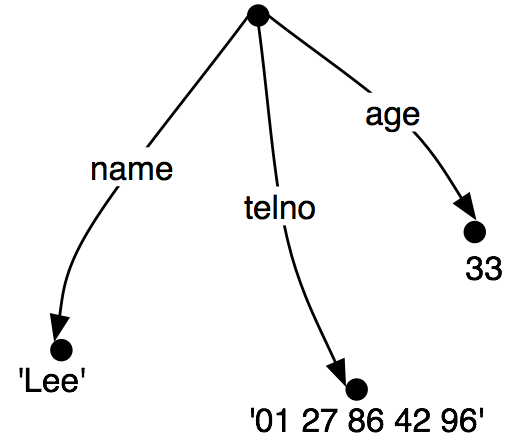The feature names appear as labels on the directed arcs, and feature values appear as labels on the nodes that are pointed to by the arcs.

Just as before, feature values can be complex:

 (36)When we look at such graphs, it is natural to think in terms of paths through the graph. A feature path is a sequence of arcs that can be followed from the root node. We will represent paths as tuples. Thus, ('address', 'street') is a feature path whose value in (36) is the string "rue Pascal".

Now let's consider a situation where Lee has a spouse named "Kim", and Kim's address is the same as Lee's. We might represent this as (37).

 (37)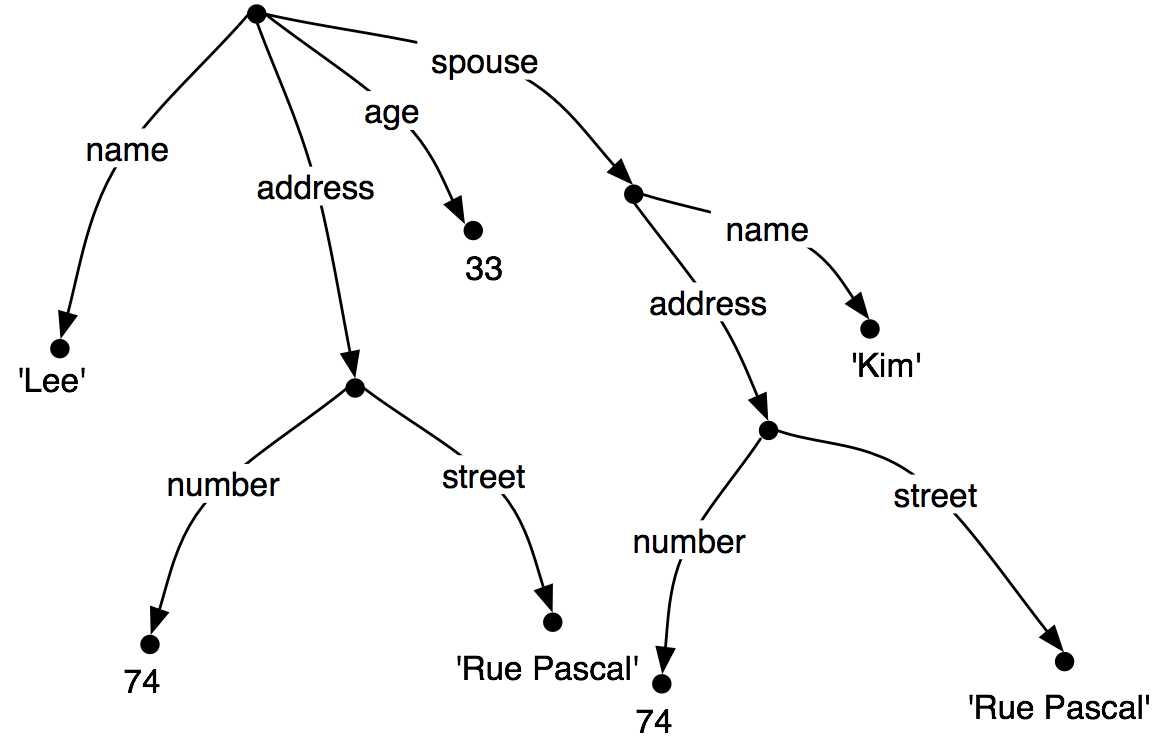However, rather than repeating the address information in the feature structure, we can "share" the same sub-graph between different arcs:

 (38)In other words, the value of the path ('ADDRESS') in (38) is identical to the value of the path ('SPOUSE', 'ADDRESS'). DAGs such as (38) are said to involve structure sharing or reentrancy. When two paths have the same value, they are said to be equivalent.

There are a number of notations for representing reentrancy in matrix-style representations of feature structures. We adopt the following convention: the first occurrence of a shared feature structure is prefixed with an integer in parentheses, such as (1), and any subsequent reference to that structure uses the notation ->(1), as shown below.

 ```>>> fs = nltk.FeatStruct("""[NAME='Lee', ADDRESS=(1)[NUMBER=74, STREET='rue Pascal'], ... SPOUSE=[NAME='Kim', ADDRESS->(1)]]""") >>> print fs [ ADDRESS = (1) [ NUMBER = 74 ] ] [ [ STREET = 'rue Pascal' ] ] [ ] [ NAME = 'Lee' ] [ ] [ SPOUSE = [ ADDRESS -> (1) ] ] [ [ NAME = 'Kim' ] ]```

(39)
 number = 74 1 street = 'rue Pascal'

name = 'Lee'
spouse =
 address → 1 name = 'Kim'

The bracketed integer is sometimes called a tag or a coindex. The choice of integer is not significant. There can be any number of tags within a single feature structure.

 `>>> fs1 = nltk.FeatStruct("[A='a', B=(1)[C='c'], D->(1), E->(1)]")`

(40)
A = 'a'
B =
 C = 'c' 1

D 1
E 1

### 10.3.3   Subsumption and Unification

It is standard to think of feature structures as providing partial information about some object, in the sense that we can order feature structures according to how general they are. For example, (41a) is more general (less specific) than (41b), which in turn is more general than (41c).

(41)

a.
 number = 74

b.
 number = 74 street = 'rue Pascal'

c.
 number = 74 street = 'rue Pascal' city = 'Paris'

This ordering is called subsumption; a more general feature structure subsumes a less general one. If FS0 subsumes FS1 (formally, we write FS0FS1), then FS1 must have all the paths and path equivalences of FS0, and may have additional paths and equivalences as well. Thus, (37) subsumes (38), since the latter has additional path equivalences.. It should be obvious that subsumption only provides a partial ordering on feature structures, since some feature structures are incommensurable. For example, (42) neither subsumes nor is subsumed by (41a).

(42)
 telno = 01 27 86 42 96

So we have seen that some feature structures are more specific than others. How do we go about specializing a given feature structure? For example, we might decide that addresses should consist of not just a street number and a street name, but also a city. That is, we might want to merge graph (43b) with (43a) to yield (43c).

(43)

 a.b.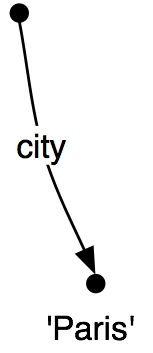c.Merging information from two feature structures is called unification and is supported by the unify() method.

 ```>>> fs1 = nltk.FeatStruct(NUMBER=74, STREET='rue Pascal') >>> fs2 = nltk.FeatStruct(CITY='Paris') >>> print fs1.unify(fs2) [ CITY = 'Paris' ] [ NUMBER = 74 ] [ STREET = 'rue Pascal' ]```

Unification is formally defined as a binary operation: FS0 FS1. Unification is symmetric, so

 (44) FS0 ⊓ FS1 = FS1 ⊓ FS0.

The same is true in Python:

 ```>>> print fs2.unify(fs1) [ CITY = 'Paris' ] [ NUMBER = 74 ] [ STREET = 'rue Pascal' ]```

If we unify two feature structures which stand in the subsumption relationship, then the result of unification is the most specific of the two:

 (45) If FS0 ⊑ FS1, then FS0 ⊓ FS1 = FS1

Unification between FS0 and FS1 will fail if the two feature structures share a path π, but the value of π in FS0 is a distinct atom from the value of π in FS1. This is implemented by setting the result of unification to be None.

 ```>>> fs0 = nltk.FeatStruct(A='a') >>> fs1 = nltk.FeatStruct(A='b') >>> fs2 = fs0.unify(fs1) >>> print fs2 None```

Now, if we look at how unification interacts with structure-sharing, things become really interesting. First, let's define (37) in Python:

 ```>>> fs0 = nltk.FeatStruct("""[NAME=Lee, ... ADDRESS=[NUMBER=74, ... STREET='rue Pascal'], ... SPOUSE= [NAME=Kim, ... ADDRESS=[NUMBER=74, ... STREET='rue Pascal']]]""")```

(46)
 number = 74 street = `rue Pascal'

name = `Lee'
spouse =
 number = 74 street = `rue Pascal'

name = `Kim'

What happens when we augment Kim's address with a specification for city? (Notice that fs1 includes the whole path from the root of the feature structure down to city.)

 `>>> fs1 = nltk.FeatStruct("[SPOUSE = [ADDRESS = [CITY = Paris]]]")`

(47) shows the result of unifying fs0 with fs1:

(47)
 number = 74 street = `rue Pascal'

name = `Lee'
spouse =
 city = `Paris' number = 74 street = `rue Pascal'

name = `Kim'

By contrast, the result is very different if fs1 is unified with the structure-sharing version fs2 (also shown as (38)):

 ```>>> fs2 = nltk.FeatStruct("""[NAME=Lee, ADDRESS=(1)[NUMBER=74, STREET='rue Pascal'], ... SPOUSE=[NAME=Kim, ADDRESS->(1)]]""")```

(48)
 city = `Paris' 1 number = 74 street = `rue Pascal'

name = `Lee'
spouse =
 address → 1 name = `Kim'

Rather than just updating what was in effect Kim's "copy" of Lee's address, we have now updated both their addresses at the same time. More generally, if a unification involves specializing the value of some path π, then that unification simultaneously specializes the value of any path that is equivalent to π.

As we have already seen, structure sharing can also be stated using variables such as ?x.

 ```>>> fs1 = nltk.FeatStruct("[ADDRESS1=[NUMBER=74, STREET='rue Pascal']]") >>> fs2 = nltk.FeatStruct("[ADDRESS1=?x, ADDRESS2=?x]") >>> print fs2 [ ADDRESS1 = ?x ] [ ADDRESS2 = ?x ] >>> print fs2.unify(fs1) [ ADDRESS1 = (1) [ NUMBER = 74 ] ] [ [ STREET = 'rue Pascal' ] ] [ ] [ ADDRESS2 -> (1) ]```

### 10.3.4   Exercises

1. ☼ Write a function subsumes() which holds of two feature structures fs1 and fs2 just in case fs1 subsumes fs2.

2. ◑ Consider the feature structures shown in Listing 10.3.

 [XX] NOTE: This example is somewhat broken -- nltk doesn't support reentrance for base feature values. (See email ~7/23/08 to the nltk-users mailing list for details.)

 ```fs1 = nltk.FeatStruct("[A = (1)b, B= [C ->(1)]]") fs2 = nltk.FeatStruct("[B = [D = d]]") fs3 = nltk.FeatStruct("[B = [C = d]]") fs4 = nltk.FeatStruct("[A = (1)[B = b], C->(1)]") fs5 = nltk.FeatStruct("[A = [D = (1)e], C = [E -> (1)] ]") fs6 = nltk.FeatStruct("[A = [D = (1)e], C = [B -> (1)] ]") fs7 = nltk.FeatStruct("[A = [D = (1)e, F = (2)[]], C = [B -> (1), E -> (2)] ]") fs8 = nltk.FeatStruct("[A = [B = b], C = [E = [G = e]]]") fs9 = nltk.FeatStruct("[A = (1)[B = b], C -> (1)]")```

Listing 10.3 (featstructures.py)

Work out on paper what the result is of the following unifications. (Hint: you might find it useful to draw the graph structures.)

1. fs1 and fs2
2. fs1 and fs3
3. fs4 and fs5
4. fs5 and fs6
5. fs7 and fs8
6. fs7 and fs9

3. ◑ List two feature structures that subsume [A=?x, B=?x].

4. ◑ Ignoring structure sharing, give an informal algorithm for unifying two feature structures.

## 10.4   Extending a Feature-Based Grammar

### 10.4.1   Subcategorization

In Chapter 7, we proposed to augment our category labels to represent different kinds of verb. We introduced labels such as iv and tv for intransitive and transitive verbs respectively. This allowed us to write productions like the following:

 (49) ```vp → iv vp → tv np ```

Although we know that iv and tv are two kinds of v, from a formal point of view iv has no closer relationship with tv than it does with np. As it stands, iv and tv are just atomic nonterminal symbols from a CFG. This approach doesn't allow us to say anything about the class of verbs in general. For example, we cannot say something like "All lexical items of category v can be marked for tense", since bark, say, is an item of category iv, not v. A simple solution, originally developed for a grammar framework called Generalized Phrase Structure Grammar (GPSG), stipulates that lexical categories may bear a subcat feature whose values are integers. This is illustrated in a modified portion of 10.1, shown in (50).

 (50) ```VP[TENSE=?t, NUM=?n] -> V[SUBCAT=0, TENSE=?t, NUM=?n] VP[TENSE=?t, NUM=?n] -> V[SUBCAT=1, TENSE=?t, NUM=?n] NP VP[TENSE=?t, NUM=?n] -> V[SUBCAT=2, TENSE=?t, NUM=?n] Sbar V[SUBCAT=0, TENSE=pres, NUM=sg] -> 'disappears' | 'walks' V[SUBCAT=1, TENSE=pres, NUM=sg] -> 'sees' | 'likes' V[SUBCAT=2, TENSE=pres, NUM=sg] -> 'says' | 'claims' V[SUBCAT=0, TENSE=pres, NUM=pl] -> 'disappear' | 'walk' V[SUBCAT=1, TENSE=pres, NUM=pl] -> 'see' | 'like' V[SUBCAT=2, TENSE=pres, NUM=pl] -> 'say' | 'claim' V[SUBCAT=0, TENSE=past, NUM=?n] -> 'disappeared' | 'walked' V[SUBCAT=1, TENSE=past, NUM=?n] -> 'saw' | 'liked' V[SUBCAT=2, TENSE=past, NUM=?n] -> 'said' | 'claimed' ```

When we see a lexical category like v[subcat 1], we can interpret the subcat specification as a pointer to the production in which v[subcat 1] is introduced as the head daughter in a vp production. By convention, there is a one-to-one correspondence between subcat values and the productions that introduce lexical heads. It's worth noting that the choice of integer which acts as a value for subcat is completely arbitrary — we could equally well have chosen 3999, 113 and 57 as our two values in (50). On this approach, subcat can only appear on lexical categories; it makes no sense, for example, to specify a subcat value on vp.

In our third class of verbs above, we have specified a category s-bar. This is a label for subordinate clauses such as the complement of claim in the example You claim that you like children. We require two further productions to analyze such sentences:

 (51) ```S-BAR -> Comp S Comp -> 'that' ```

The resulting structure is the following.

 (52)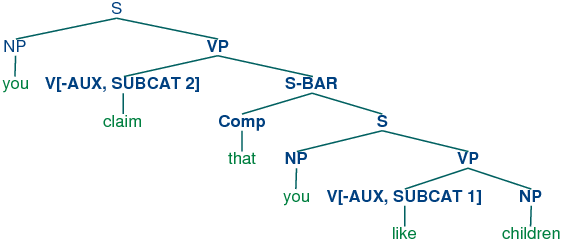An alternative treatment of subcategorization, due originally to a framework known as categorial grammar, is represented in feature-based frameworks such as PATR and Head-driven Phrase Structure Grammar. Rather than using subcat values as a way of indexing productions, the subcat value directly encodes the valency of a head (the list of arguments that it can combine with). For example, a verb like put that takes np and pp complements (put the book on the table) might be represented as (53):

 (53) v[subcat 〈np, np, pp〉 ]

This says that the verb can combine with three arguments. The leftmost element in the list is the subject np, while everything else — an np followed by a pp in this case — comprises the subcategorized-for complements. When a verb like put is combined with appropriate complements, the requirements which are specified in the subcat are discharged, and only a subject np is needed. This category, which corresponds to what is traditionally thought of as vp, might be represented as follows.

 (54) v[subcat 〈np〉 ]

Finally, a sentence is a kind of verbal category that has no requirements for further arguments, and hence has a subcat whose value is the empty list. The tree (55) shows how these category assignments combine in a parse of Kim put the book on the table.

 (55)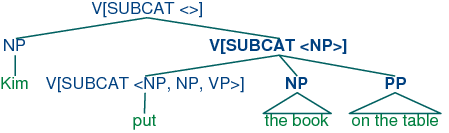X-bar syntax (cf. [Jacobs & Rosenbaum, 1970], [Jackendoff, 1977]) addresses this issue by abstracting out the notion of phrasal level. It is usual to recognize three such levels. If n represents the lexical level, then n' represents the next level up, corresponding to the more traditional category Nom, while n'' represents the phrasal level, corresponding to the category np. (The primes here replace the typographically more demanding horizontal bars of [Jacobs & Rosenbaum, 1970]). (56) illustrates a representative structure.

 (56)(57)The productions in (58) illustrate how bar levels can be encoded using feature structures.

 (58) ```s → n[bar=2] v[bar=2] n[bar=2] → Det n[bar=1] n[bar=1] → n[bar=1] p[bar=2] n[bar=1] → n[bar=0] p[bar=2] ```

### 10.4.3   Auxiliary Verbs and Inversion

Inverted clauses — where the order of subject and verb is switched — occur in English interrogatives and also after 'negative' adverbs:

(59)

 a. Do you like children?

 b. Can Jody walk?

(60)

 a. Rarely do you see Kim.

 b. Never have I seen this dog.

However, we cannot place just any verb in pre-subject position:

(61)

 a. *Like you children?

 b. *Walks Jody?

(62)

 a. *Rarely see you Kim.

 b. *Never saw I this dog.

Verbs that can be positioned initially in inverted clauses belong to the class known as auxiliaries, and as well as do, can and have include be, will and shall. One way of capturing such structures is with the following production:

 (63) ```S[+inv] -> V[+AUX] NP VP ```

 (64)### 10.4.4   Unbounded Dependency Constructions

Consider the following contrasts:

(65)

 a. You like Jody.

 b. *You like.

(66)

 a. You put the card into the slot.

 b. *You put into the slot.

 c. *You put the card.

 d. *You put.

The verb like requires an np complement, while put requires both a following np and pp. Examples (65) and (66) show that these complements are obligatory: omitting them leads to ungrammaticality. Yet there are contexts in which obligatory complements can be omitted, as (67) and (68) illustrate.

(67)

 a. Kim knows who you like.

 b. This music, you really like.

(68)

 a. Which card do you put into the slot?

 b. Which slot do you put the card into?

That is, an obligatory complement can be omitted if there is an appropriate filler in the sentence, such as the question word who in (67a), the preposed topic this music in (67b), or the wh phrases which card/slot in (68). It is common to say that sentences like (67)(68) contain gaps where the obligatory complements have been omitted, and these gaps are sometimes made explicit using an underscore:

(69)

 a. Which card do you put __ into the slot?

 b. Which slot do you put the card into __?

(70)

 a. *Kim knows who you like Jody.

 b. *This music, you really like hip-hop.

(71)

 a. *Which card do you put this into the slot?

 b. *Which slot do you put the card into this one?

The mutual co-occurence between filler and gap leads to (67)(68) is sometimes termed a "dependency". One issue of considerable importance in theoretical linguistics has been the nature of the material that can intervene between a filler and the gap that it licenses; in particular, can we simply list a finite set of strings that separate the two? The answer is No: there is no upper bound on the distance between filler and gap. This fact can be easily illustrated with constructions involving sentential complements, as shown in (72).

(72)

 a. Who do you like __?

 b. Who do you claim that you like __?

 c. Who do you claim that Jody says that you like __?

Since we can have indefinitely deep recursion of sentential complements, the gap can be embedded indefinitely far inside the whole sentence. This constellation of properties leads to the notion of an unbounded dependency construction; that is, a filler-gap dependency where there is no upper bound on the distance between filler and gap.

 (73)The top part of the tree introduces the filler who (treated as an expression of category np[+wh]) together with a corresponding gap-containing constituent s/np. The gap information is then "percolated" down the tree via the vp/np category, until it reaches the category np/np. At this point, the dependency is discharged by realizing the gap information as the empty string e immediately dominated by np/np.

Do we need to think of slash categories as a completely new kind of object in our grammars? Fortunately, no, we don't — in fact, we can accommodate them within our existing feature-based framework. We do this by treating slash as a feature, and the category to its right as a value. In other words, our "official" notation for s/np will be s[slash=NP]. Once we have taken this step, it is straightforward to write a small grammar for analyzing unbounded dependency constructions. 10.4 illustrates the main principles of slash categories, and also includes productions for inverted clauses. To simplify presentation, we have omitted any specification of tense on the verbs.

 ```>>> nltk.data.show_cfg('grammars/feat1.fcfg') % start S # ############################ # Grammar Rules # ############################ S[-INV] -> NP S/NP S[-INV]/?x -> NP VP/?x S[+INV]/?x -> V[+AUX] NP VP/?x S-BAR/?x -> Comp S[-INV]/?x NP/NP -> VP/?x -> V[SUBCAT=1, -AUX] NP/?x VP/?x -> V[SUBCAT=2, -AUX] S-BAR/?x VP/?x -> V[SUBCAT=3, +AUX] VP/?x # ############################ # Lexical Rules # ############################ V[SUBCAT=1, -AUX] -> 'see' | 'like' V[SUBCAT=2, -AUX] -> 'say' | 'claim' V[SUBCAT=3, +AUX] -> 'do' | 'can' NP[-WH] -> 'you' | 'children' | 'girls' NP[+WH] -> 'who' Comp -> 'that'```

Listing 10.4 (slashcfg.py): Grammar for Simple Long-distance Dependencies

The grammar in Listing 10.4 contains one gap-introduction production, namely

 (74) ```s[-inv] → np s/np ```

In order to percolate the slash feature correctly, we need to add slashes with variable values to both sides of the arrow in productions that expand s, vp and np. For example,

 (75) ```vp/?x → v s-bar/?x ```

says that a slash value can be specified on the vp mother of a constituent if the same value is also specified on the s-bar daughter. Finally, (76) allows the slash information on np to be discharged as the empty string.

 (76) ```np/np → ```

Using 10.4, we can parse the string who do you claim that you like into the tree shown in (77).

 (77)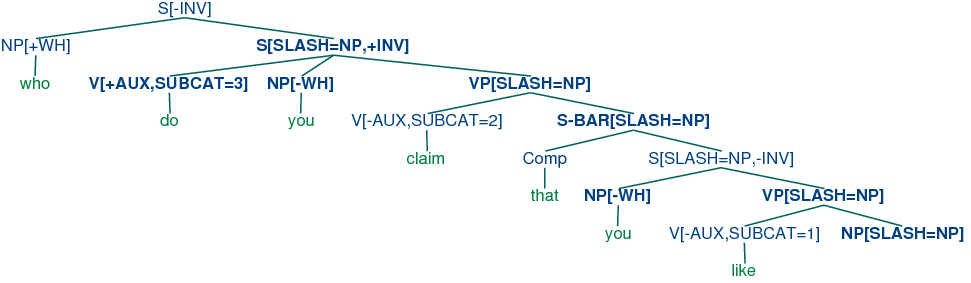### 10.4.5   Case and Gender in German

Compared with English, German has a relatively rich morphology for agreement. For example, the definite article in German varies with case, gender and number, as shown in Table 10.2.

Table 10.2:

Morphological Paradigm for the German definite Article

 Case Masc Fem Neut Plural Nom der die das die Gen des der des der Dat dem der dem den Acc den die das die

Subjects in German take the nominative case, and most verbs govern their objects in the accusative case. However, there are exceptions like helfen that govern the dative case:

(78)

a.
 Die Katze sieht den Hund the.NOM.FEM.SG cat.3.FEM.SG see.3.SG the.ACC.MASC.SG dog.3.MASC.SG 'the cat sees the dog'

b.
 *Die Katze sieht dem Hund the.NOM.FEM.SG cat.3.FEM.SG see.3.SG the.DAT.MASC.SG dog.3.MASC.SG

c.
 Die Katze hilft dem Hund the.NOM.FEM.SG cat.3.FEM.SG help.3.SG the.DAT.MASC.SG dog.3.MASC.SG 'the cat helps the dog'

d.
 *Die Katze hilft den Hund the.NOM.FEM.SG cat.3.FEM.SG help.3.SG the.ACC.MASC.SG dog.3.MASC.SG

The grammar 10.5 illustrates the interaction of agreement (comprising person, number and gender) with case.

 ```>>> nltk.data.show_cfg('grammars/german.fcfg') % start S # Grammar Rules S -> NP[CASE=nom, AGR=?a] VP[AGR=?a] NP[CASE=?c, AGR=?a] -> PRO[CASE=?c, AGR=?a] NP[CASE=?c, AGR=?a] -> Det[CASE=?c, AGR=?a] N[CASE=?c, AGR=?a] VP[AGR=?a] -> IV[AGR=?a] VP[AGR=?a] -> TV[OBJCASE=?c, AGR=?a] NP[CASE=?c] # Lexical Rules # Singular determiners # masc Det[CASE=nom, AGR=[GND=masc,PER=3,NUM=sg]] -> 'der' Det[CASE=dat, AGR=[GND=masc,PER=3,NUM=sg]] -> 'dem' Det[CASE=acc, AGR=[GND=masc,PER=3,NUM=sg]] -> 'den' # fem Det[CASE=nom, AGR=[GND=fem,PER=3,NUM=sg]] -> 'die' Det[CASE=dat, AGR=[GND=fem,PER=3,NUM=sg]] -> 'der' Det[CASE=acc, AGR=[GND=fem,PER=3,NUM=sg]] -> 'die' # Plural determiners Det[CASE=nom, AGR=[PER=3,NUM=pl]] -> 'die' Det[CASE=dat, AGR=[PER=3,NUM=pl]] -> 'den' Det[CASE=acc, AGR=[PER=3,NUM=pl]] -> 'die' # Nouns N[AGR=[GND=masc,PER=3,NUM=sg]] -> 'hund' N[CASE=nom, AGR=[GND=masc,PER=3,NUM=pl]] -> 'hunde' N[CASE=dat, AGR=[GND=masc,PER=3,NUM=pl]] -> 'hunden' N[CASE=acc, AGR=[GND=masc,PER=3,NUM=pl]] -> 'hunde' N[AGR=[GND=fem,PER=3,NUM=sg]] -> 'katze' N[AGR=[GND=fem,PER=3,NUM=pl]] -> 'katzen' # Pronouns PRO[CASE=nom, AGR=[PER=1,NUM=sg]] -> 'ich' PRO[CASE=acc, AGR=[PER=1,NUM=sg]] -> 'mich' PRO[CASE=dat, AGR=[PER=1,NUM=sg]] -> 'mir' PRO[CASE=nom, AGR=[PER=2,NUM=sg]] -> 'du' PRO[CASE=nom, AGR=[PER=3,NUM=sg]] -> 'er' | 'sie' | 'es' PRO[CASE=nom, AGR=[PER=1,NUM=pl]] -> 'wir' PRO[CASE=acc, AGR=[PER=1,NUM=pl]] -> 'uns' PRO[CASE=dat, AGR=[PER=1,NUM=pl]] -> 'uns' PRO[CASE=nom, AGR=[PER=2,NUM=pl]] -> 'ihr' PRO[CASE=nom, AGR=[PER=3,NUM=pl]] -> 'sie' # Verbs IV[AGR=[NUM=sg,PER=1]] -> 'komme' IV[AGR=[NUM=sg,PER=2]] -> 'kommst' IV[AGR=[NUM=sg,PER=3]] -> 'kommt' IV[AGR=[NUM=pl, PER=1]] -> 'kommen' IV[AGR=[NUM=pl, PER=2]] -> 'kommt' IV[AGR=[NUM=pl, PER=3]] -> 'kommen' TV[OBJCASE=acc, AGR=[NUM=sg,PER=1]] -> 'sehe' | 'mag' TV[OBJCASE=acc, AGR=[NUM=sg,PER=2]] -> 'siehst' | 'magst' TV[OBJCASE=acc, AGR=[NUM=sg,PER=3]] -> 'sieht' | 'mag' TV[OBJCASE=dat, AGR=[NUM=sg,PER=1]] -> 'folge' | 'helfe' TV[OBJCASE=dat, AGR=[NUM=sg,PER=2]] -> 'folgst' | 'hilfst' TV[OBJCASE=dat, AGR=[NUM=sg,PER=3]] -> 'folgt' | 'hilft'```

Listing 10.5 (germancfg.py): Example Feature-Based Grammar

As you will see, the feature objcase is used to
specify the case that the verb governs on its object.

### 10.4.6   Exercises

1. ☼ Modify the grammar illustrated in (50) to incorporate a bar feature for dealing with phrasal projections.

2. ☼ Modify the German grammar in 10.5 to incorporate the treatment of subcategorization presented in 10.4.1.

3. ◑ Extend the German grammar in 10.5 so that it can handle so-called verb-second structures like the following:

 (79) Heute sieht der hund die katze.

4. ★ Morphological paradigms are rarely completely regular, in the sense of every cell in the matrix having a different realization. For example, the present tense conjugation of the lexeme walk only has two distinct forms: walks for the 3rd person singular, and walk for all other combinations of person and number. A successful analysis should not require redundantly specifying that 5 out of the 6 possible morphological combinations have the same realization. Propose and implement a method for dealing with this.

5. ★ So-called head features are shared between the mother and head daughter. For example, tense is a head feature that is shared between a vp and its head v daughter. See [Gazdar et al, 1985] for more details. Most of the features we have looked at are head features — exceptions are subcat and slash. Since the sharing of head features is predictable, it should not need to be stated explicitly in the grammar productions. Develop an approach that automatically accounts for this regular behavior of head features.

## 10.5   Summary

• The traditional categories of context-free grammar are atomic symbols. An important motivation feature structures is to capture fine-grained distinctions that would otherwise require a massive multiplication of atomic categories.
• By using variables over feature values, we can express constraints in grammar productions that allow the realization of different feature specifications to be inter-dependent.
• Typically we specify fixed values of features at the lexical level and constrain the values of features in phrases to unify with the corresponding values in their daughters.
• Feature values are either atomic or complex. A particular sub-case of atomic value is the Boolean value, represented by convention as [+/- f].
• Two features can share a value (either atomic or complex). Structures with shared values are said to be re-entrant. Shared values are represented by numerical indices (or tags) in AVMs.
• A path in a feature structure is a tuple of features corresponding to the labels on a sequence of arcs from the root of the graph representation.
• Two paths are equivalent if they share a value.
• Feature structures are partially ordered by subsumption. FS0 subsumes FS1 when FS0 is more general (less informative) than FS1.
• The unification of two structures FS0 and FS1, if successful, is the feature structure FS2 that contains the combined information of both FS0 and FS1.
• If unification specializes a path π in FS, then it also specializes every path π' equivalent to π.
• We can use feature structures to build succinct analyses of a wide variety of linguistic phenomena, including verb subcategorization, inversion constructions, unbounded dependency constructions and case government.

For more examples of feature-based parsing with NLTK, please see the guides at http://nltk.org/doc/guides/featgram.html, http://nltk.org/doc/guides/featstruct.html, and http://nltk.org/doc/guides/grammartestsuites.html.

For an excellent introduction to the phenomenon of agreement, see [Corbett, 2006].

The earliest use of features in theoretical linguistics was designed to capture phonological properties of phonemes. For example, a sound like /b/ might be decomposed into the structure [+labial, +voice]. An important motivation was to capture generalizations across classes of segments; for example, that /n/ gets realized as /m/ preceding any +labial consonant. Within Chomskyan grammar, it was standard to use atomic features for phenomena like agreement, and also to capture generalizations across syntactic categories, by analogy with phonology. A radical expansion of the use of features in theoretical syntax was advocated by Generalized Phrase Structure Grammar (GPSG; [Gazdar et al, 1985]), particularly in the use of features with complex values.

Coming more from the perspective of computational linguistics, [Dahl & Saint-Dizier, 1985] proposed that functional aspects of language could be captured by unification of attribute-value structures, and a similar approach was elaborated by [Grosz & Stickel, 1983] within the PATR-II formalism. Early work in Lexical-Functional grammar (LFG; [Bresnan, 1982]) introduced the notion of an f-structure that was primarily intended to represent the grammatical relations and predicate-argument structure associated with a constituent structure parse. [Shieber, 1986] provides an excellent introduction to this phase of research into feature-based grammars.

One conceptual difficulty with algebraic approaches to feature structures arose when researchers attempted to model negation. An alternative perspective, pioneered by [Kasper & Rounds, 1986] and [Johnson, 1988], argues that grammars involve descriptions of feature structures rather than the structures themselves. These descriptions are combined using logical operations such as conjunction, and negation is just the usual logical operation over feature descriptions. This description-oriented perspective was integral to LFG from the outset (cf. [Huang & Chen, 1989], and was also adopted by later versions of Head-Driven Phrase Structure Grammar (HPSG; [Sag & Wasow, 1999]). A comprehensive bibliography of HPSG literature can be found at http://www.cl.uni-bremen.de/HPSG-Bib/.

Feature structures, as presented in this chapter, are unable to capture important constraints on linguistic information. For example, there is no way of saying that the only permissible values for num are sg and pl, while a specification such as [num=masc] is anomalous. Similarly, we cannot say that the complex value of agr must contain specifications for the features per, num and gnd, but cannot contain a specification such as [subcat=3]. Typed feature structures were developed to remedy this deficiency. To begin with, we stipulate that feature values are always typed. In the case of atomic values, the values just are types. For example, we would say that the value of num is the type num. Moreover, num is the most general type of value for num. Since types are organized hierarchically, we can be more informative by specifying the value of num is a subtype of num, namely either sg or pl.

In the case of complex values, we say that feature structures are themselves typed. So for example the value of agr will be a feature structure of type agr. We also stipulate that all and only per, num and gnd are appropriate features for a structure of type agr. A good early review of work on typed feature structures is [Emele & Zajac, 1990]. A more comprehensive examination of the formal foundations can be found in [Carpenter, 1992], while [Copestake, 2002] focuses on implementing an HPSG-oriented approach to typed feature structures.

There is a copious literature on the analysis of German within feature-based grammar frameworks. [Nerbonne, Netter, & Pollard, 1994] is a good starting point for the HPSG literature on this topic, while [M{\"u}ller, 2002] gives a very extensive and detailed analysis of German syntax in HPSG.

Chapter 15 of [Jurafsky & Martin, 2008] discusses feature structures, the unification algorithm, and the integration of unification into parsing algorithms.